# #优质创作者# 随机梯度下降法的数学基础 原创

0收藏

【本文正在参加优质创作者激励计划】

## 导数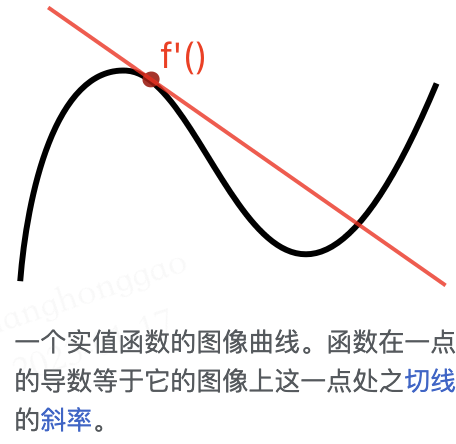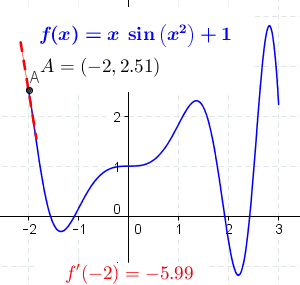$$\lim _{x\to a}{\frac {f(x)-f(a)}{x-a}}}$$

## 偏导数

$$z = f(x, y) = x^2 + xy + y^2$$

$${\frac {\partial f}{\partial x}} = 2x + y$$

$${{\frac {\partial f}{\partial y}} = x + 2y}$$

## 方向导数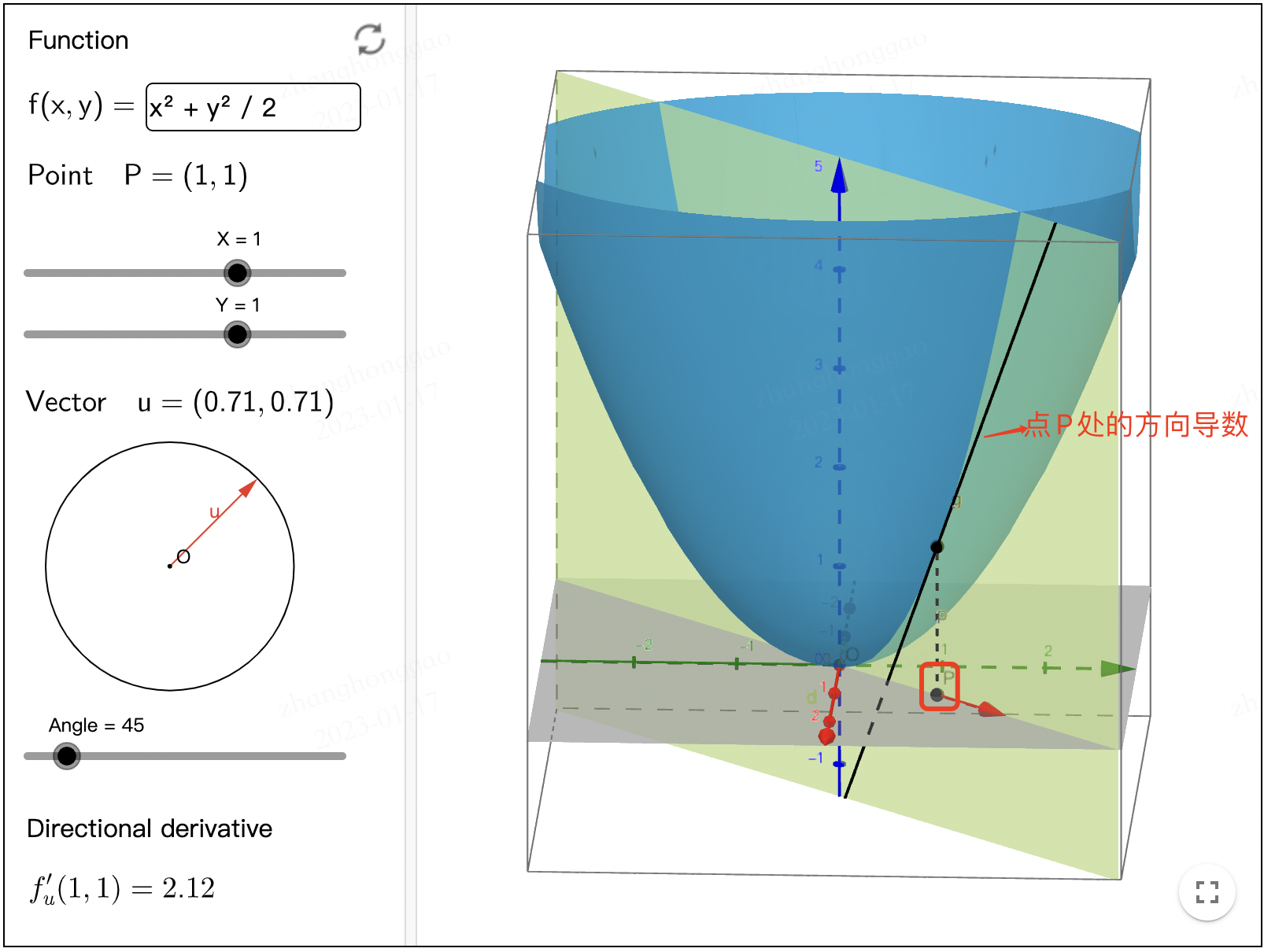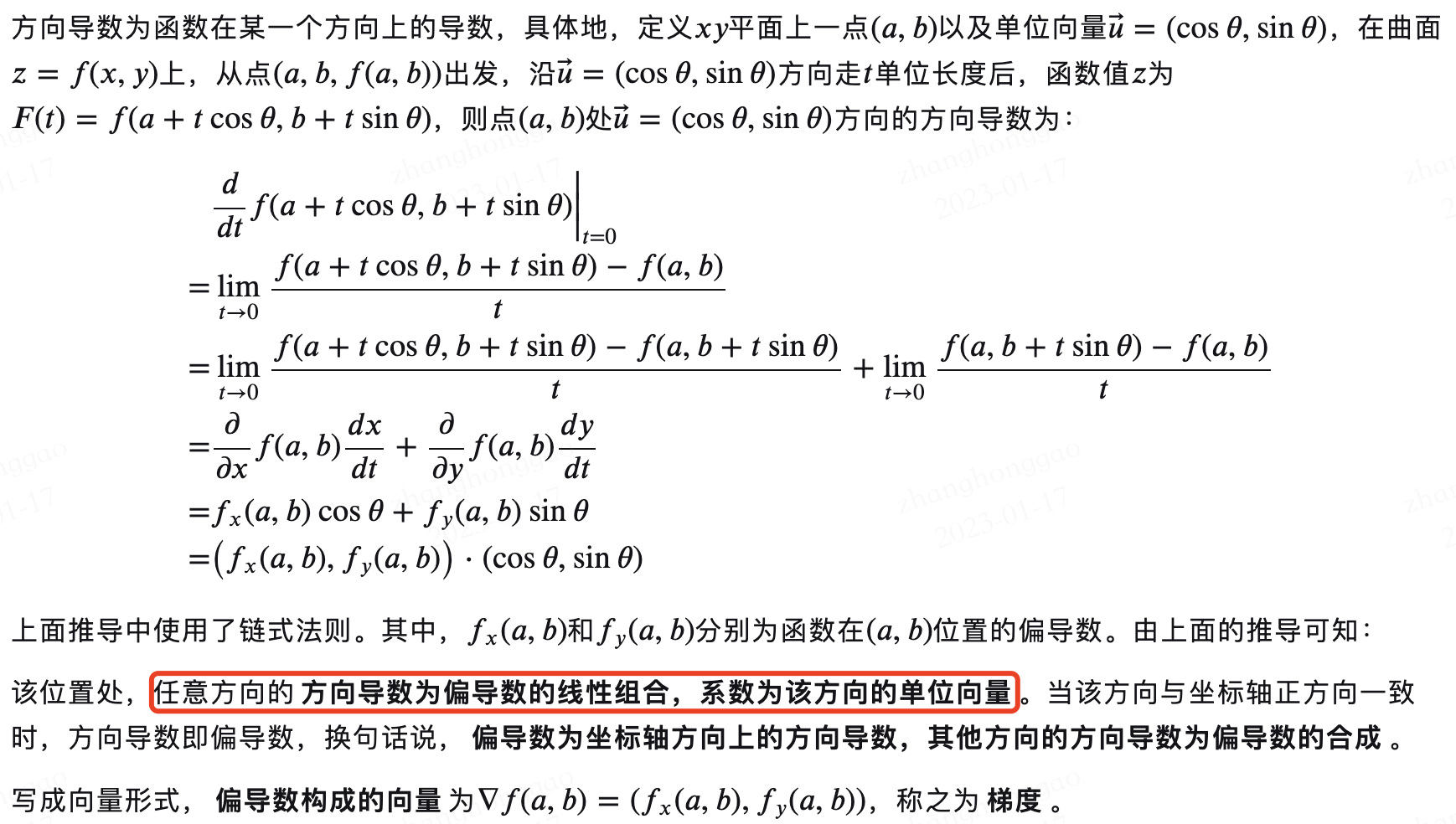## 梯度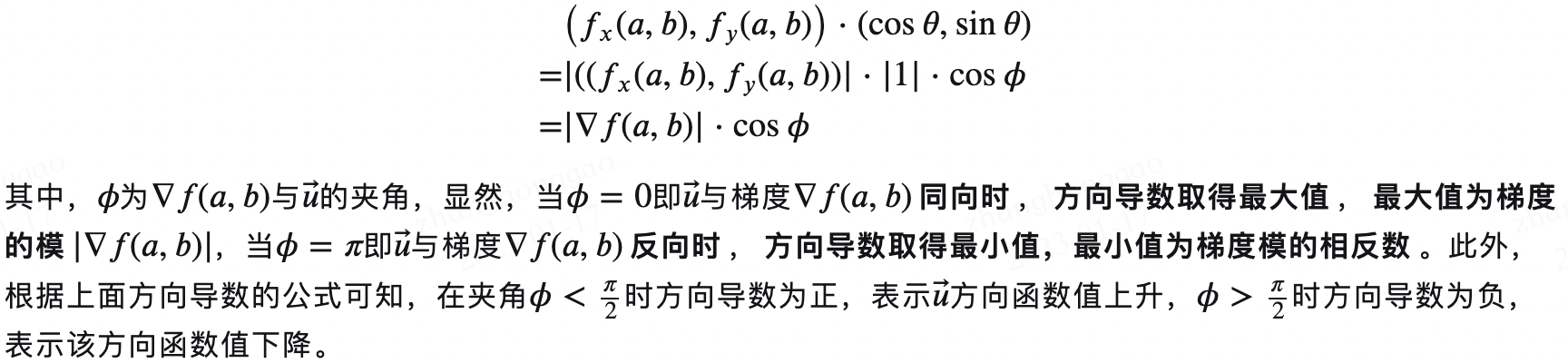1. 假设有一个房间，房间内所有点的温度由一个标量场 $\phi$ 给出的，即点 $(x,y,z)$ 的温度是 $\phi(x,y,z)$。假设温度不随时间改变。然后，在房间的每一点，该点的梯度将显示变热最快的方向。梯度的大小将表示在该方向上的温度变化率。

2. 考虑一座高度函数为 $H$ 的山，山上某点 $(x, y)$ 的高度是 $H(x, y)$，点 $(x,y)$ 的梯度是在该点坡度（或者说斜度）最陡的方向。梯度的大小会告诉我们坡度到底有多陡。

• 当前位置的梯度方向，为函数在该位置处方向导数最大的方向，也是函数值上升最快的方向，反方向为下降最快的方向；
• 当前位置的梯度长度（模），为最大方向导数的值。

## 总结

• 方向导数是各个方向上的导数。
• 偏导数连续才有梯度存在。
• 偏导数构成的向量为梯度。
• 梯度的方向是方向导数中取到最大值的方向，梯度的值是方向导数的最大值。

## 参考资料